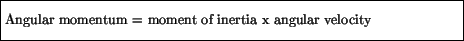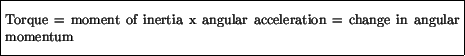Next: Questions Up: Rotational Motion Previous: Rotational kinetic energy

# Angular momentum

Recall for linear motion that we introduced the concept of (linear) momentum, mass x velocity, by equating the force exerted on an object to the change in the object's momentum. An analogous quantity called angular momentum can be introduced in the same way. For an object rotating about an axis, the angular momentum is defined aswhich leads to the net torque exerted on the object being expressed asConservation of linear momentum is a powerful tool in analyzing, for example, collisions between objects undergoing linear motion. Similarly, conservation of angular momentum is very useful in many situations. Consider again the ice skater spinning around. If she draws her arms inwards, she decreases her moment of inertia. Conservation of angular momentum requires that the skater's moment of inertia times her angular velocity remain constant. Thus if her moment of inertia decreases, her angular velocity must increase, and this is what we observe: when the skater pulls in her arms, she immediately starts to rotate faster.

It is also worth noting that just like linear momentum, conservation of angular momentum is associated with a symmetry of the laws of physics. In this case, the relevant symmetry has to do with rotations about any axis.Next: Questions Up: Rotational Motion Previous: Rotational kinetic energy
modtech@theory.uwinnipeg.ca
1999-09-29